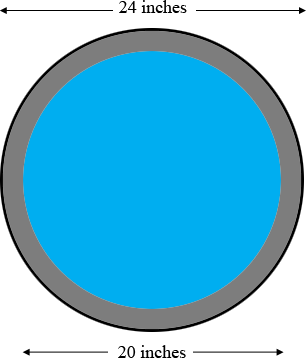SEARCH HOMEMath Central Quandaries & QueriesQuestion from jeanne: I need 2 inches of cement around a 20 inch pipe that is 20 feet long. Thank you.Hi Jeanne,

Looking at the end of the pipe, this is what I see.Imagine that you fill the entire cylinder which is 24 inches in diameter and 20 feet long with concrete. You can use our volume calculator to calculate how much concrete you would need.

Now imagine that you drilled a 20 inch diameter hole down the centre of the tube you just created. Use our volume calculator to calculate the amount of concrete you removed.

The difference is the amount of concrete that remains.

PennyMath Central is supported by the University of Regina and The Pacific Institute for the Mathematical Sciences.